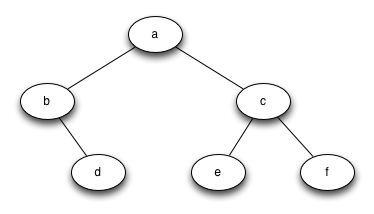# 6.5. List of Lists Representation¶

In a tree represented by a list of lists, we will begin with Python’s list data structure and write the functions defined above. Although writing the interface as a set of operations on a list is a bit different from the other abstract data types we have implemented, it is interesting to do so because it provides us with a simple recursive data structure that we can look at and examine directly. In a list of lists tree, we will store the value of the root node as the first element of the list. The second element of the list will itself be a list that represents the left subtree. The third element of the list will be another list that represents the right subtree. To illustrate this storage technique, let’s look at an example. Figure 1 shows a simple tree and the corresponding list implementation.

my_tree = [
"a",  # root
["b",  # left subtree
["d", [], []],
["e", [], []]
],
["c",  # right subtree
["f", [], []],
[]
],
]


Notice that we can access subtrees of the list using standard list indexing. The root of the tree is my_tree, the left subtree of the root is my_tree, and the right subtree is my_tree. ActiveCode 1 illustrates creating a simple tree using a list. Once the tree is constructed, we can access the root and the left and right subtrees. One very nice property of this list of lists approach is that the structure of a list representing a subtree adheres to the structure defined for a tree; the structure itself is recursive! A subtree that has a root value and two empty lists is a leaf node. Another nice feature of the list of lists approach is that it generalizes to a tree that has many subtrees. In the case where the tree is more than a binary tree, another subtree is just another list.

Let’s formalize this definition of the tree data structure by providing some functions that make it easy for us to use lists as trees. Note that we are not going to define a binary tree class. The functions we will write will just help us manipulate a standard list as though we are working with a tree.

def make_binary_tree(root):
return [root, [], []]


The make_binary_tree function simply constructs a list with a root node and two empty sublists for the children. To add a left subtree to the root of a tree, we need to insert a new list into the second position of the root list. We must be careful, however. If the list already has something in the second position, we need to keep track of it and push it down the tree as the left child of the list we are adding. Listing 1 shows the Python code for inserting a left child.

Listing 1

def insert_left(root, new_child):
old_child = root.pop(1)
if len(old_child) > 1:
root.insert(1, [new_child, old_child, []])
else:
root.insert(1, [new_child, [], []])
return root


Notice that to insert a left child, we first obtain the (possibly empty) list that corresponds to the current left child. We then add the new left child, installing the old left child as the left child of the new one. This allows us to splice a new node into the tree at any position. The code for insert_right is similar to insert_left and is shown in Listing 2.

Listing 2

def insert_right(root, new_child):
old_child = root.pop(2)
if len(old_child) > 1:
root.insert(2, [new_child, [], old_child])
else:
root.insert(2, [new_child, [], []])
return root


To round out this set of tree-making functions, let’s write a couple of access functions for getting and setting the root value, as well as getting the left or right subtrees. These functions can be seen in Listing 3.

Listing 3

def get_root_val(root):
return root

def set_root_val(root, new_value):
root = new_value

def get_left_child(root):
return root

def get_right_child(root):
return root


ActiveCode 2 makes use of the tree functions we have just written. You should try it out for yourself. One of the exercises at the end of this chapter asks you to draw the tree structure resulting from this set of calls.

Self Check

Write a function build_tree that returns a tree using the list of lists functions that looks like this: